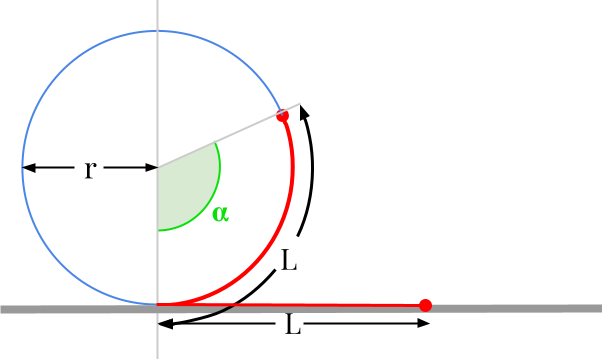Question #5729

# How to convert meters to degrees?

Merged questions

You can't actually convert meters to degrees. Degree is a measure of angle, while meter is a unit of distance. There is an homogeneity issue between the two quantities.However, there are many mechanical systems that transform linear motion into rotary motion. The most obvious system is the wheel: if you move forward the vehicle, the wheels turn and vice versa. In this case, the formula governing such a system and allowing to transform a displacement given in meters into a rotation of an angle expressed in degrees is given by:

﻿﻿

﻿﻿

with :

• ﻿﻿ the distance or length expressed in metres [m]
• ﻿﻿ is the radius expressed in metres [m]
• ﻿﻿ is the rotation angle expressed in degrees [deg]

Example: Take the example of a vehicle equipped with wheels with a radius of 0.25 m (50 cm in diameter). Assume the vehicle is moving 10 meters forward. We can calculate the angle of rotation of the wheels:

﻿﻿

If we divide 2281° by 360°, we can conclude that the vehicle wheels has made about 6.3 revolutions.

6 events in history

Icons proudly provided by Friconix.